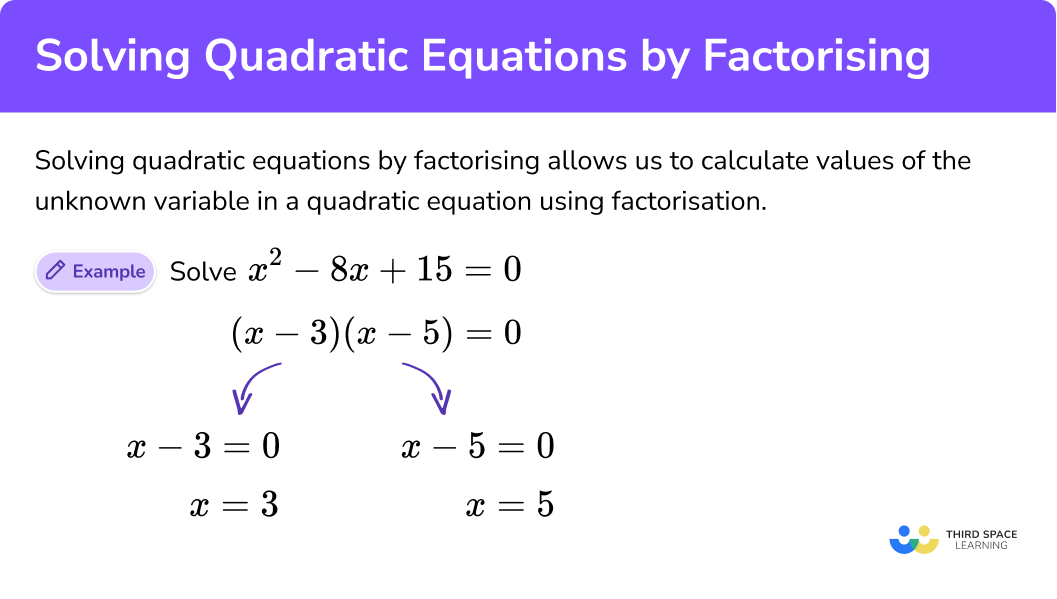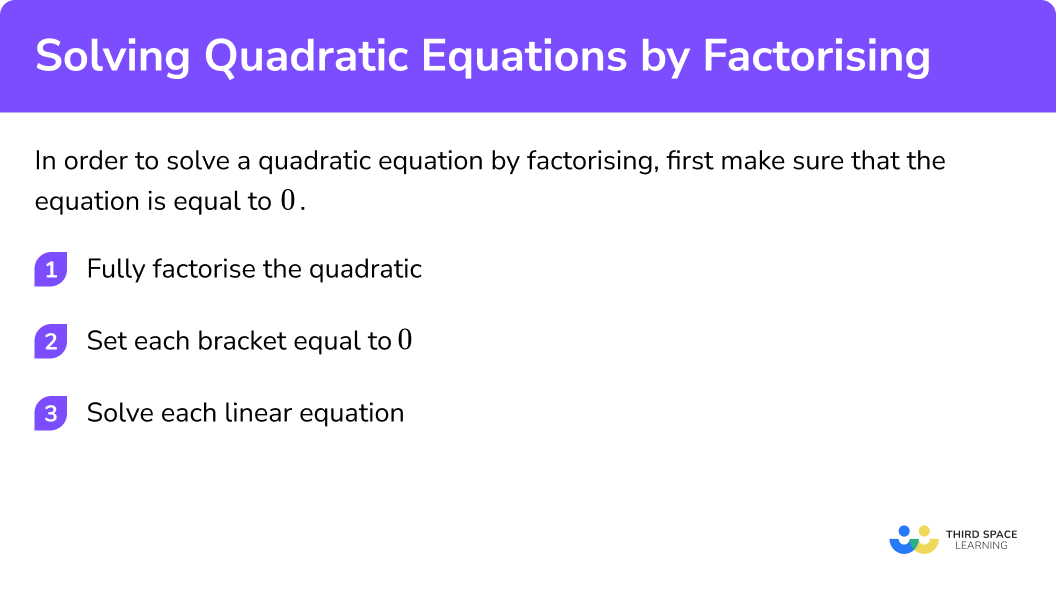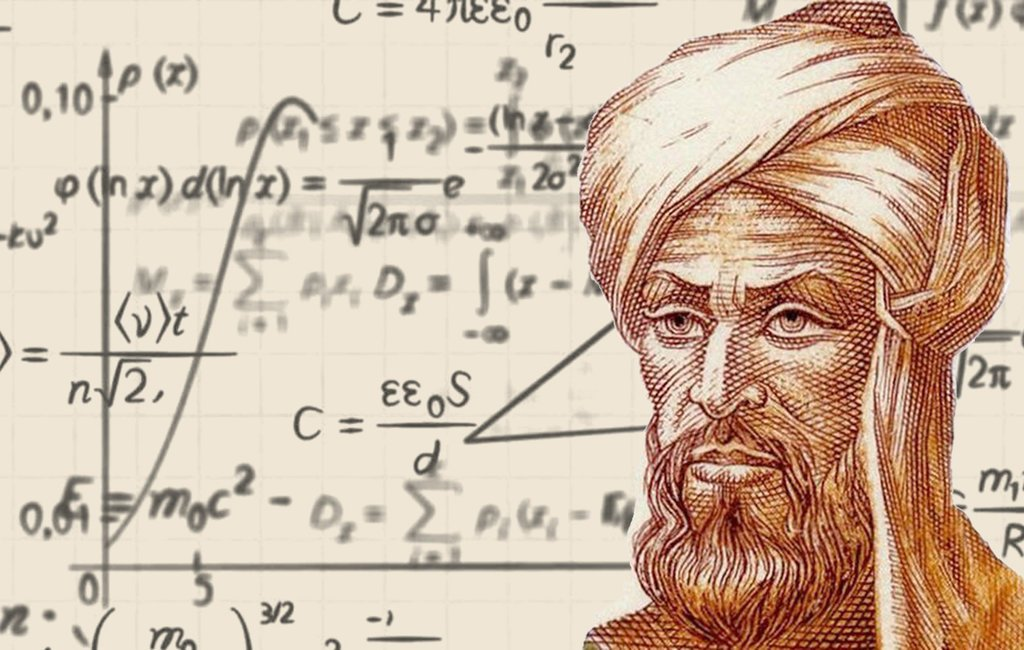GCSE Maths Algebra Equations Solving Equations Quadratic Equations

# Solving Quadratic Equations By Factorising

Here we will learn about solving quadratic equations by factorising including how to solve quadratic equations by factorising when a = 1 and when a > 1.
There are also solving quadratic equations worksheets based on Edexcel, AQA and OCR exam questions, along with further guidance on where to go next if you’re still stuck.

## What is solving quadratic equations by factorising?

Solving quadratic equations by factorising allows us to calculate values of the unknown variable in a quadratic equation using factorisation.

To do this we make sure the equation is equal to 0, factorise it into brackets and then solve the resulting linear equations.

E.g.

Solve the following quadratic equation by factorising:

$x^{2}-2x-15 =0$
\begin{align} x^{2} – 2x – 15 &= 0 \\ (x-5)(x + 3) &= 0 \end{align}

So,

\begin{align} x – 5&=0 \\ x &= 5 \end{align}

and

\begin{align} x + 3 &= 0 \\ x &= -3 \end{align}

E.g.

Solve the following quadratic equation by factorising:

$2 x^2+7 x – 4 = 0$
\begin{align} 2x^{2} + 7x – 4 &= 0 \\ (2x – 1)(x + 4) &= 0 \end{align}

So,

\begin{align} 2 x – 1&=0 \\ x &= \frac{1}{2} \end{align}

and

\begin{align} x + 4 &= 0 \\ x &= -4 \end{align}

### What is solving quadratic equations by factorising?## How to solve quadratic equations

In order to factor a quadratic algebraic equation we need to make sure it is in the form of the general quadratic equation:

$ax^{2}+bx+c=0$

We must ensure the quadratic equation is equal to 0, rearranging it if necessary.

NOTE: Quadratic equations are a type of polynomial equation because they consist of two or more algebraic terms.

## How to solve a quadratic equation by factorising

First make sure that the equation is equal to 0.

Step 1: Fully factorise the quadratic.

Step 2: Set each bracket equal to 0.

Step 3: Solve each linear equation.

Step-by-step guide: Solving linear equations

### Explain how to solve a quadratic equation by factorising in 3 steps### Related lessons on quadratic equations

Solving quadratic equations by factorising is part of our series of lessons to support revision on quadratic equations and solving equations. You may find it helpful to start with the main solving equations lesson for a summary of what to expect, or use the step by step guides below for further detail on individual topics. Other lessons in this series include:

## Examples of solving quadratic equations

### Example 1: solve a quadratic equation by factorising when a = 1

Solve

$x^{2}-8x+15=0$

1. Fully factorise the quadratic equation.
$(x-3)(x-5)=0$

2We know that if two values multiply together to get 0, at least one of them must be 0. So set each bracket equal to 0.

$x-3=0\qquad \qquad x-5=0$

3Solve each equation to find x.

The opposite of -3 is +3, so +3 to both sides of the equation.

The opposite of -5 is +5, so +5 on left hand side, and on the right hand side.

When we solve a quadratic equation we normally have two solutions.

We call these the solutions, or roots, of the quadratic equation.

When we plot these values on an x, y grid we get a special ‘U’ shaped curve called a parabola.

We can see the real roots of the quadratic equation are where the quadratic graph crosses the x-axis.

We can check that our solution is correct by substituting it into the original equation.

### Example 2: solve a quadratic equation by factorising when a > 1

Solve

$2x^{2}+5x+3=0$

$2x+3=0 \qquad \qquad x+1=0$

The opposite of +3 is -3, so -3 to both sides of the equation. The opposite of × 2 is ÷ 2, so ÷ 2 on the left hand side, and on the right hand side.

The opposite of +1 is -1, so -1 to both sides of the equation.

When we solve a quadratic equation we normally have two solutions.

We call these the solutions or roots of the quadratic equation.

We can see the real roots of the quadratic equation are where the quadratic graph crosses the x-axis.

We can check that our solution is correct by substituting it into the quadratic function.

### Common misconceptions

• Solve by factorising into two brackets
E.g.
\begin{aligned} x^{2}-2x-24&=0\\ (x-6)(x+4)&=0 \end{aligned}

Do not try and square root the quadratic otherwise you will not get all the solutions!

• The order of the brackets doesn’t matter

When we multiply two values the order doesn’t matter.

E.g.

$2\times 3=3\times 2$

It is exactly the same here:

$(x-6)(x+4)$

means

$(x-6)\times (x+4)$

So,

$(x-6)(x+4)=0$

is the same as

$(x+4)(x-6)=0$

• Forgetting to solve after factorising

Don’t  forget to set the factorised expression equal to zero and solve it.

Always check you have answered the question.

• Factorising or factoring?

The term factorising can sometimes be written as ‘factoring’ or ‘factorization’.

• Difference of two squares

If the quadratic equation involves two square terms (perfect squares) that are subtracted from each other, you will need to use the ‘difference of two squares’ to factorise it.

$4 x^{2}-16=0 \\ (2 x+4)(2 x-4)=0 \\ 2 x+4=0, \quad 2 x-4=0$

• Equation cannot be factorised

If a quadratic equation cannot be factorised, we can still solve it by using the quadratic formula.

To work out the number of real solutions a quadratic equation has we can use the discriminant. The derivation of the quadratic formula is fascinating, we will explore it more when we learn about ‘completing  the square’.

• Equation should be in the below form before trying to factorise
$a x^{2}+b x+c=0$

It is possible to factorise a quadratic equation without ensuring that it is in the standard form of a quadratic equation, however this can be challenging.

• When we solve a quadratic equation by factorisation at GCSE we will always get real numbers that are rational numbers

### Practice solving quadratic equations questions

1. Solve: {x}^2+5x+6=0

x=3      x=-2x=3      x=2x=-3     x=-2x=-3     x=2{x}^2+5x+6=0 can be factorised as (x+2)(x+3)=0 . Setting each bracket equal to zero and solving leads to the required solutions.2. Solve: {x}^2-x-20=0

x=-5     x=-4x=5      x=4x=-5     x=4x=5      x=-4{x}^2-x-20=0 can be factorised as (x-5)(x+4)=0 . Setting each bracket equal to zero and solving leads to the required solutions.3. Solve: 2{x}^2+3x-9=02{x}^2+3x-9=0 can be factorised as (2x-3)(x+3)=0 . Setting each bracket equal to zero and solving leads to the required solutions.4. Solve: 3{x}^2-9x+6=0

x=2     x=1x=-2     x=-1x=2      x=-1x=-2    x=13{x}^2-9x+6=0 can be factorised as 3(x-1)(x-2)=0 . Setting each bracket equal to zero and solving leads to the required solutions.### Solving quadratic equations GCSE questions

1. Factorise x^{2}-x-30

(2 marks)

(x+5)  or  (x-6)

(1)

(x+5) (x-6)

(1)

2. Hence, or otherwise, solve x^{2}-x-30=0

(1 mark)

 x=-5  and  x=6

(1)

3. Solve 2 x^{2}-5 x-3

(3 marks)

(2x+1) (x-3)=0

(1)

2x+1=0 and x-3=0

(1)

x=-\frac{1}{2} \text { and } x=3

(1)

### Did you know?

• Did you know that Al-Khwarizmi (Abu Ja’far Muhammad ibn Musa al-Khwarizmi) was one of the first people in history to write about algebra? He lived in Baghdad in around 780 to 850 AD and he wrote a book called “Hisab Al-jabr w’al-muqabala”, in which we get the word ‘algebra’ (meaning ‘restoration of broken parts’) from.Copyright (c) 2021 by khaled (https://codepen.io/ksawalme/pen/xLvGOv)

• Did you know the ancient babylonians could solve quadratic equations using a method equivalent to the quadratic formula, despite not using algebraic notation!
• Did you also know that the ancient Greek mathematician Euclid used geometric methods to solve quadratic equations way back in 300BC! His book, “The Elements”, is one of the most studied books in human history.

The history of mathematics is amazing!

## Learning checklist

You have now learned how to:

• Solve quadratic equations algebraically by factorising
• Solve quadratic equations by finding approximate solutions using a graph

## Still stuck?

Prepare your KS4 students for maths GCSEs success with Third Space Learning. Weekly online one to one GCSE maths revision lessons delivered by expert maths tutors.

Find out more about our GCSE maths tuition programme.